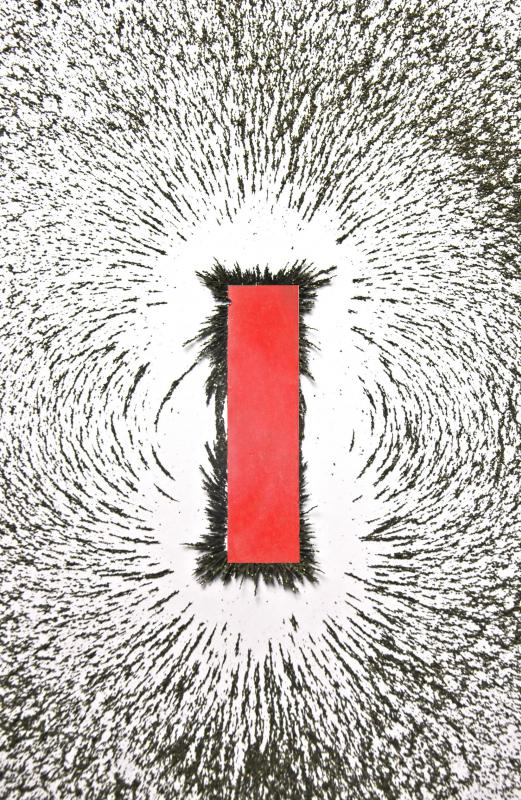# What is Magnetomotive Force?

Treasure Ingels-Thompson

When physicists discuss the force of a magnetic field, they refer to it as magnetomotive force (mmf) or magnetic potential. Magnetomotive force is the work that carries a measurable unit of strength through a magnetic circuit. This unit of strength is measured in ampere-turns (AT).Bar magnet with iron filings to illustrate the magnetic field.

Magnetism in a circuit flows from the north to the south pole. Following a specific path, the force of the magnetism is similar to the force in an electrical circuit. While electricity follows a certain path from a point of origin to a receiving point and back, magnetism always flows from one pole to the other. As magnetism flows through the circuit, it produces lines of force, or flux lines, and generates a magnetic field.

In simplest terms, anything that produces magnetism can be described as exerting mmf. Understanding the basic meaning of the word itself makes it easy to understand its application. Magnetomotive force translates, quite literally, to "magnetic motion causing." The motion that the force generates is always exerted perpendicular to the magnetic circuit's resistance.

Although magnetism exerts a force all its own, it does exhibit a reluctance to travel across large spans of air. This reluctance is similar to the resistance of an electrical circuit. Magnetism travels much more successfully through iron. A magnetic circuit composed exclusively of iron will have much lower reluctance than a magnetic circuit crosses an air gap.

Either permanent magnets or coiled wire conducting electricity may produce a magnetomotive force. When a coil of wire produces a magnetomotive force, the number of turns of the wire is directly related to the value of the force generated. For instance, the magnetic motion force of a coil with 50 turns would be 25 times greater than the magnetomotive force of a coil with only two turns. The magnetic flux of a magnetic circuit is, then, equal to the magnet motion force divided by the circuit's reluctance. This relationship may be better understood if one takes time to consider that magnetic flux represents the journey of a substance's magnetism through a magnetic circuit. Each turn in a coil concentrates the force of the magnetic. Overcoming the reluctance of the circuit to travel through each of the coils, the natural strength of the magnet is demonstrated as sheer force.

## You might also Likeanon168211

Is this force (magnetomotive force), related to Newton or kilogram units, or what?

JavaGhoul